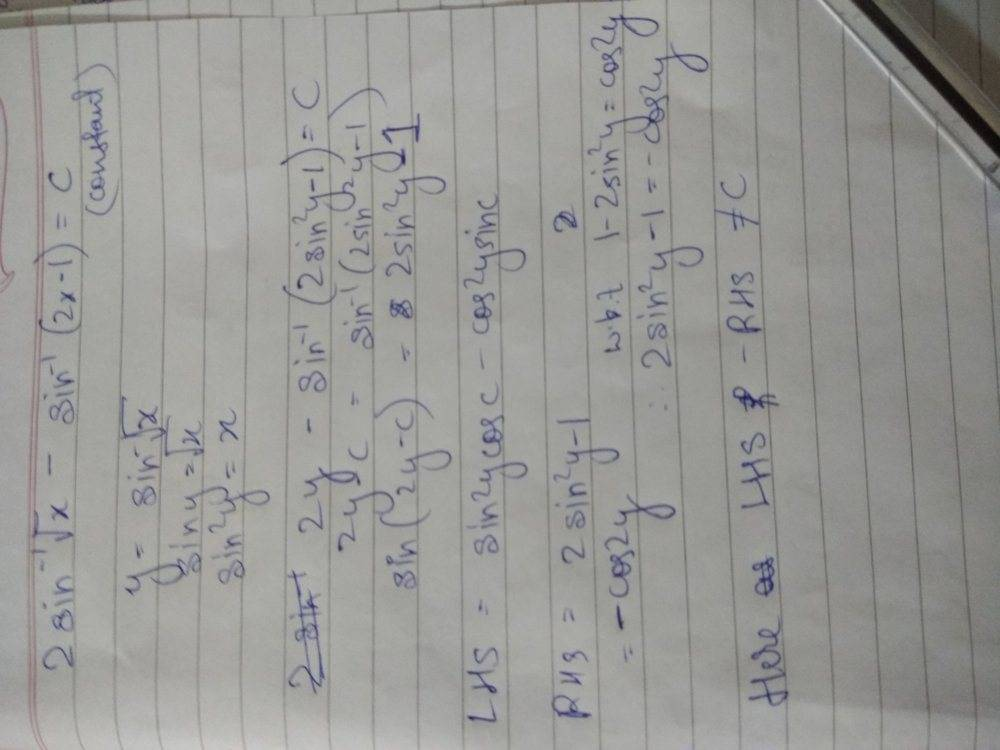# Indefinite Integration

Crystal037
Homework Statement:
Integrate 1/(x(1-x))^(1/2)dx
Relevant Equations:
Integral of 1/rt(1-x^2)dx = arcsinx
Let x=t^2
Then dx=2t dt
Integral of 1/(x(1-x))^(1/2)dx
= integral of 2tdt/t(1-t^2) ^(1/2)
= integral of 2dt/(1-t^2) ^(1/2)
= 2 arcsin(t) +c
= 2 arcsin(rt(x)) +c.
But the answer in my book is arcsin(2x-1) +c.
Tell me how
2 arcsin(rt(x) +C= arcsin(2x-1) +c
I know the constant will vary for both the answers and both the answers must come equal after some manipulation. Is my answer correct.

Gold Member
Try to prove that ##2 arcsin(x)-arcsin(2x-1)## is a constant.
Just denote be ##y=arcsin x## and then ##2x-1 = 2\sin y -1##, so you need to show that the following is constant: ##2y-arcsin(2\sin y -1)##, if you denote this by ##u## you get: ##2y-u=arcsin(2\sin y -1)## which means that: ##\sin (2y-u) = 2\sin(y)-1##; from here you need to solve a trig equation like in high school.

Gold Member
I meant ##y = arcsin \sqrt{x}##.

Homework Helper
Gold Member
2022 Award
Homework Statement:: Integrate 1/(x(1-x))^(1/2)dx
Relevant Equations:: Integral of 1/rt(1-x^2)dx = arcsinx

Let x=t^2
Then dx=2t dt
Integral of 1/(x(1-x))^(1/2)dx
= integral of 2tdt/t(1-t^2) ^(1/2)
= integral of 2dt/(1-t^2) ^(1/2)
= 2 arcsin(t) +c
= 2 arcsin(rt(x)) +c.
But the answer in my book is arcsin(2x-1) +c.
Tell me how
2 arcsin(rt(x) +C= arcsin(2x-1) +c
I know the constant will vary for both the answers and both the answers must come equal after some manipulation. Is my answer correct.
One good thing about doing integration is that you can always check your answer by differentiating it to check you get back the integrand.

I don't understand, however, this variable ##r## that seems to come and go from one line to the next.

Crystal037
One good thing about doing integration is that you can always check your answer by differentiating it to check you get back the integrand.

I don't understand, however, this variable ##r## that seems to come and go from one line to the next.
There is no variable r I used rt to denote root

Homework Helper
Gold Member
2022 Award
•JD_PM
Crystal037
One good thing about doing integration is that you can always check your answer by differentiating it to check you get back the integrand.

I don't understand, however, this variable ##r## that seems to come and go from one line to the next.
Yeah I differentiated it and the answer is correct. Thank you

Crystal037I am getting LHS as a function of cos2y and sin 2y while RHS only as a function of cos2y. Where am I getting wrong

#### Attachments

Homework Helper
Gold Member
2022 Award
I am getting LHS as a function of cos2y and sin 2y while RHS only as a function of cos2y. Where am I getting wrong?
The quickest way must be to differentiate, surely? If the derivative is zero, then the function is a constant. And, setting ##x =0## will give you the constant.

Otherwise, you need the double angle formula.

Homework Helper
Gold Member
For integrating, even more simple I think just substitution in original x = t + ½ .

•SammyS and archaic
Staff Emeritus
Homework Helper
Gold Member
Using a graphing calculator you can find that ##\displaystyle 2\arcsin(\sqrt{x}\ ) - \arcsin(2x-1) ## is ##\dfrac{\pi}{2} ## .

This suggests that ##\displaystyle 2\arcsin(\sqrt{x}\ ) - \dfrac{\pi}{2} = \arcsin(2x-1) ##.

Take the sine of the left hand side and you get ##2x-1##, after a bit of manipulation.

archaic
$$\int \frac{1}{\sqrt{x(1-x)}} dx=\int\frac{1}{\sqrt x}\cdot\frac{1}{\sqrt{1-x}} dx$$